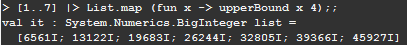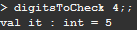# Project Euler – Problem 30 Solution

Check out my new course Learn you some Lambda best practice for great good! and learn the best practices for performance, cost, security, resilience, observability and scalability.

#### Problem

Surprisingly there are only three numbers that can be written as the sum of fourth powers of their digits:

1634 = 14 + 64 + 34 + 44

8208 = 84 + 24 + 04 + 84

9474 = 94 + 44 + 74 + 44

As 1 = 14 is not a sum it is not included.

The sum of these numbers is 1634 + 8208 + 9474 = 19316.

Find the sum of all the numbers that can be written as the sum of fifth powers of their digits.

#### Solution

```// get the digits of a number into an array
let getDigits (n:bigint) = n.ToString().ToCharArray() |> Array.map (fun c -> bigint.Parse(c.ToString()))

// get the sum of a number's digits to the specified power
let getDigitsToPowerSum (n:bigint) pow =
getDigits(n) |> Array.map (fun x -> pown x pow) |> Array.sum

// get the max sum achievable by a number of the given number of digits to the specified power
let upperBound (digits:int) pow = bigint(digits) * pown 9I pow

// find the last number of digits n, where the max sum achievable by the digits of a number of
// n digits to the specified power is greater than the smallest number of n digits
// any number with more than n digits do not need to be checked
let digitsToCheck pow =
let n =
Seq.unfold (fun state -> Some(state, (state+1))) 1
|> Seq.filter (fun x -> (upperBound x pow).ToString().ToCharArray().Length < x)
n-1

// get the next number with the given number of digits
let maxNumber digits = [1..digits] |> List.map (fun x -> 9I * pown 10I (x-1)) |> List.sum

let max = maxNumber (digitsToCheck 5)
[2I..max] |> List.filter (fun n -> n = getDigitsToPowerSum n 5) |> List.sum
```

One of the tricky things with this problem is that there’s no upper limit to how far you’d need to test before you can safely determine that you’ve tested all the numbers whose sum of fifth powers of their digits MIGHT be equal to the numbers themselves.

But that’s not to say it’s not possible, for a 1-digit number, i.e. 1..9, the max sum of its digits is 1 * (9 POW 5) = 59049; similarly for a 2-digit number the max sum is 2 * (9 POW 5) = 118098. The upperBound function calculates this upper ceiling for a number of a given digits and power.

Let’s take a closer look at the distribution of this upper limit as the number of digits go up, for the fourth power:so as you can see, when the number of digits reaches 6, the max sum of fourth powers of digits is 39366, but the smallest 6-digit number is 100000! Therefore it’s impossible for any 6 digit number to be written as the sum of fourth powers of its digits, and it means we’ll need to go as far as covering all 5 digit numbers. The digitsToCheck function simply encapsulates this bit of logic:The maxNumber function is a helper function which generates the biggest number of the given number of digits. To find the answer, I simply had to make use of all the helper functions mentioned so far and sum the numbers whose fifth powers of its digits equals itself.Hi, I’m Yan. I’m an AWS Serverless Hero and the author of Production-Ready Serverless.

I specialise in rapidly transitioning teams to serverless and building production-ready services on AWS.

Are you struggling with serverless or need guidance on best practices? Do you want someone to review your architecture and help you avoid costly mistakes down the line? Whatever the case, I’m here to help.Check out my new course, Learn you some Lambda best practice for great good! In this course, you will learn best practices for working with AWS Lambda in terms of performance, cost, security, scalability, resilience and observability. Enrol now and enjoy a special preorder price of £9.99 (~\$13).Are you working with Serverless and looking for expert training to level-up your skills? Or are you looking for a solid foundation to start from? Look no further, register for my Production-Ready Serverless workshop to learn how to build production-grade Serverless applications!